# 数学与生活–时钟的原理

• A+

## 基础数学知识

1. 我们都知道1s = 1 / 60min,1min = 1 / 60h;

2. 观察任意一款手表或时钟，我们会发现，通常时钟的表盘会被分成12个大格，512=60个小格，而整个表盘是360°，也就是说每个小格是6°。换句话说，秒针和分针每动一下，都会走过1小格，走过了6°，而时针动一下，则走过了一大格，56°=30°；因此，秒针和分针移动的基值是6°，时针是30°；

3. 另外在分针走动的时候，时针也不是静止不动的，而是不甘寂寞的默默向前移动，也就是说当分针动一下，走了6°时，时针实际上也挪动了1 / 60 * 30°。所以，计算时针角度时不要忽略了分针对其的影响。（虽然秒针对分针也有着同样的影响，但几乎看不出来，所以，通常我们会将其忽略）

## 确定各指针的角度

``````sdegree = - nSeconds * 6 + 90;

mdegree = - nMinutes * 6 + 90;

hdegree =- ( (nHour%12) * 30 + Math.floor((nMinutes/60)*30) ) + 90;

（-90，是因为rotateZ角度旋转规则，默认是从水平开始，逆时针为+，顺时针为-）
``````

### 图形解释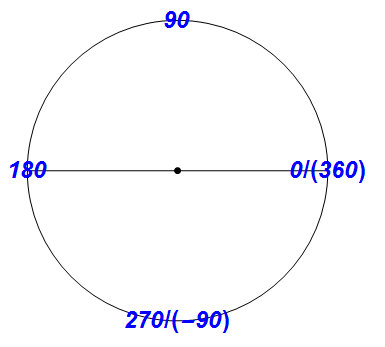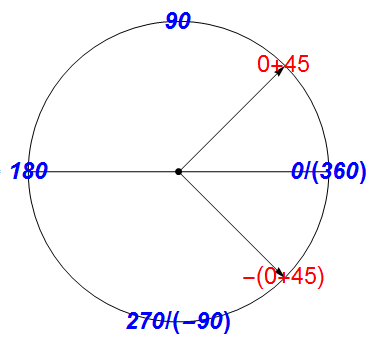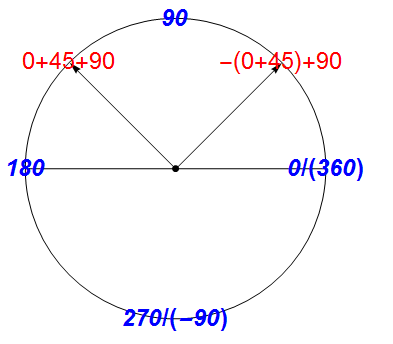## mathematica编程

### 自己编写

``````DynamicModule[{t = (t = None)},

Column[{
Dynamic[
"Update Time:", {"On" :> (t = (UpdateInterval -> 1);),
"Off" :> (t = None;)}]],

Dynamic[
Refresh[
x = TimeObject[];
Graphics[{
{Dashed, Red, Circle[]},(*画出表盘*)
Black, PointSize[Large], Point[{0, 0}],(*加入表盘中间的一个点*)
{Text[Style["12", Bold, Blue, Italic, 24], {0, 1}],
Text[Style["3", Bold, Blue, Italic, 24], {1, 0}],
Text[Style["6", Bold, Blue, Italic, 24], {0, -1}],
Text[Style["9", Bold, Blue, Italic, 24], {-1, 0}]},(*画出钟上的数字*)

Text[x, {0, -.5}],(*下方显示具体时间*)
Arrow[{{0, 0}, {Cos[(-(DateList[x][[-1]]*6) + 90) Degree],Sin[(-(DateList[x][[-1]]*6) + 90) Degree]}}],(*模拟秒针的走动*)
Arrow[{{0,0}, .7*{Cos[(-(DateList[x][[-2]]*6) + 90) Degree], Sin[(-(DateList[x][[-2]]*6) + 90) Degree]}}],(*模拟分针的走动*)
Arrow[{{0, 0}, .5*{Cos[(-(Mod[DateList[x][[-3]], 12]*30 + Floor[DateList[x][[-2]]/2]) + 90) Degree], Sin[(-(Mod[DateList[x][[-3]], 12]*30 +Floor[DateList[x][[-2]]/2]) +90) Degree]}}](*模拟时针的走动*)
}], t]
]}, Center]
]
``````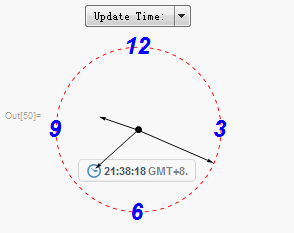### 使用自带函数--ClockGauge

mathematica真的是函数太全了，竟然还有专门画表盘的函数`ClockGauge`，下面我们来改进一下代码。

``````DynamicModule[{t = (t = None)},
Column[{
Dynamic[
"Update Time:", {"On" :> (t = (UpdateInterval -> 1);),
"Off" :> (t = None;)}]],
Dynamic[
Refresh[
ClockGauge[AbsoluteTime[],
PlotLabel -> Style["当地时间", Large, Bold]], t]
]
}, Center
]
]``````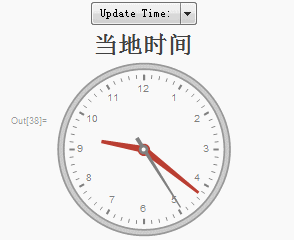## 参考资料

• 微信公众号
• 关注微信公众号
•• QQ群
• 我们的QQ群号
•